It is always nice to have tidy and visually appealing graphs.

As a matter of fact there is always a few lines of code necessary to beautify default graphs. This post contains those handy snippets and even more.

There are few ways to follow:

• tune matplotlib-plotted graphs: dejunkify, set color scheme, additional tweaks
• seaborn library with predefined scheme + a few more tunes via library wrapper

### Dejunkify matplotlib graph

First, import matplotlib, instantiate variables and plot graph.

``````import matplotlib.pyplot as plt
import numpy as np
%matplotlib notebook

# Define Variables
train_score = [ 0.56647847, 0.93155951, 0.99039881, 1., 1., 1. ]
test_score = [ 0.56768547, 0.92959558, 0.98965952, 1., 0.99507994, 0.52240279 ]
ind = np.arange(6)
width = 0.3

# Barplots
fig, ax = plt.subplots(figsize=(10,8))
bars_train = ax.bar(ind, train_score, width, linewidth = 0.3, edgecolor='black', alpha = 0.8, label='Train');
bars_test = ax.bar(ind+width, test_score, width, linewidth = 0.3, edgecolor='black', alpha = 0.8, label='Test');
``````
``````<IPython.core.display.Javascript object>
``````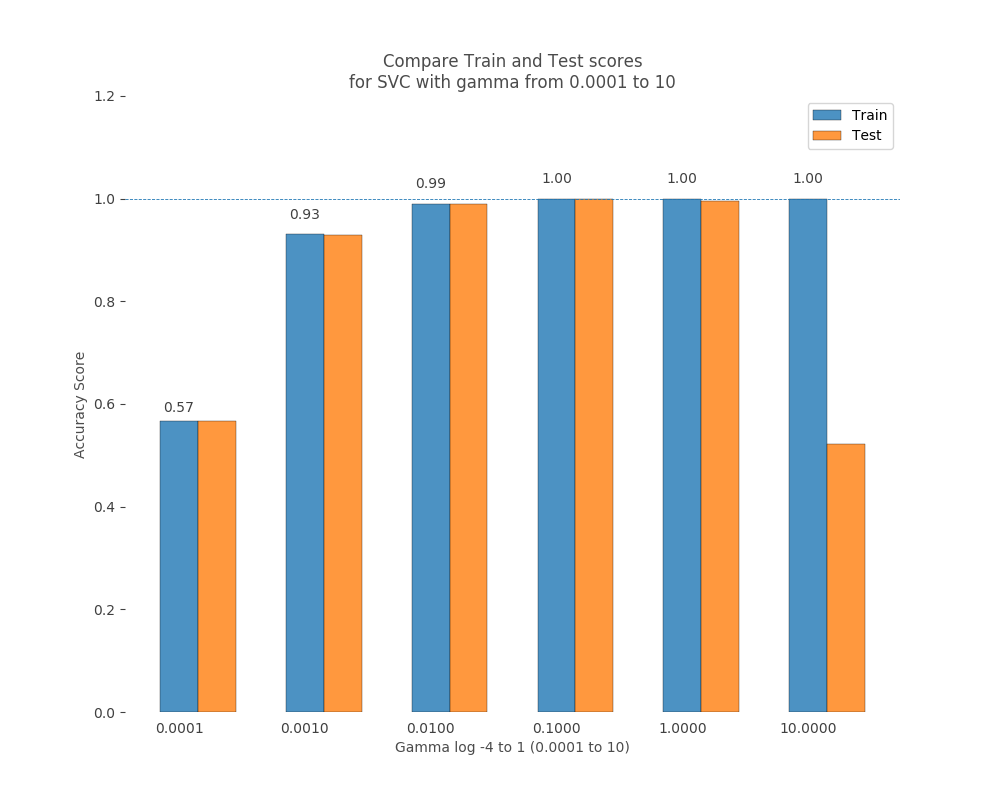#### Aesthetic code snippet

Now improve visual part.

Setting horizontal line, title, labels, limits, remove spines and unnecessary ticks, assigns ticks and ticklabels we need, show the legend, and also soften text.

``````# Improve Aesthetics
ax.axhline(1, lw=0.6, linestyle='--')

ax.set_title('Compare Train and Test scores\nfor SVC with gamma from 0.0001 to 10', alpha=0.7)
ax.set_ylim(0, 1.2)
ax.set_ylabel('Accuracy Score', alpha = 0.7)
ax.set_xlabel('Gamma log -4 to 1 (0.0001 to 10)', alpha = 0.7)
ax.set_xticks(ind)
ax.set_xticklabels(['0.0001', '0.0010', '0.0100', '0.1000', '1.0000', '10.0000'])

ax.tick_params(axis='both', top='off', bottom='off', left='on', right='off',
labelleft='on', labelbottom='on',
color='#404040',
labelcolor='#404040')
for i,spine in enumerate(ax.spines.values()):
spine.set_visible(False)

ax.legend();
``````
``````### Adding values atop the bars:
for bar in bars_train:
height = bar.get_height()
print(height)
ax.text(bar.get_x() + bar.get_width()/2, (height+height*0.03), '{:.2f}'.format(float(height)),
ha='center', color='black', fontsize=10, alpha=0.75)
``````
``````0.56647847
0.93155951
0.99039881
1.0
1.0
1.0
``````

### Layout and annotations

We can use a very distinctive color palette ColorBlind 10. Color palettes on Tableau.

``````cb_dark_blue = (0/255, 107/255, 164/255)
cb_orange = (255/255, 128/255, 14/255)

# Apply color and linewidth as following:
ax.plot(x, y, c=cb_dark_blue, linewidth=3)
ax.plot(x, y, c=cb_orange, linewidth=3)

ax.text(x, y, 'Vivid label')
``````

## Seaborn

Seaborn visualization library, which is built on top of matplotlib. Seaborn has good support for more complex plots, attractive default styles, and integrates well with the pandas library.

Even importing seaborn automatically affects all graphs.

``````import seaborn as sns
import pandas as pd

# Define data
cols = ['Survived', 'Pclass', 'Sex', 'Age', 'SibSp', 'Parch', 'Fare', 'Embarked']
titanic = titanic[cols].dropna()
``````

#### distplot

Histogram with linear contour

``````# Plotting histogram via seaborn
plt.figure()
sns.distplot(titanic['Age'])
plt.show()
``````
``````<IPython.core.display.Javascript object>
``````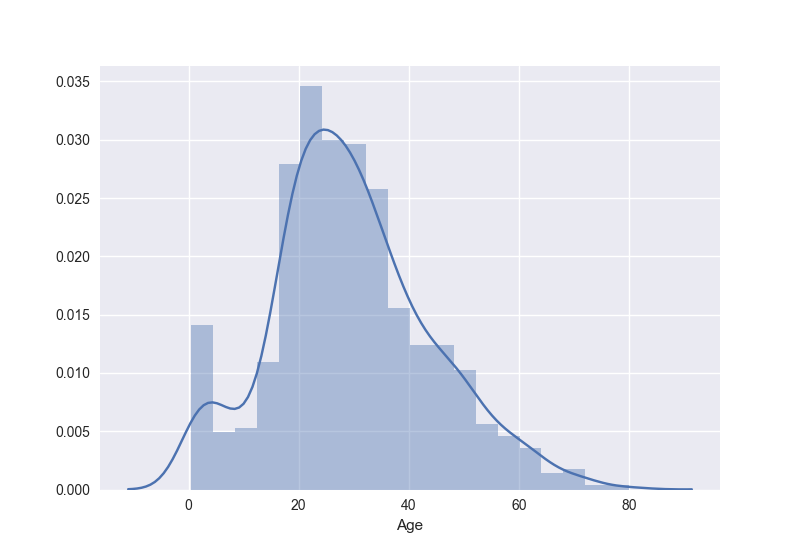#### Seaborn styling

Predefined styles can be chosen with `seaborn.set_style()` function:

• `darkgrid`: Coordinate grid displayed, dark background color
• `whitegrid`: Coordinate grid displayed, white background color
• `dark`: Coordinate grid hidden, dark background color
• `white`: Coordinate grid hidden, white background color
• `ticks`: Coordinate grid hidden, white background color, ticks visible
``````plt.figure()

sns.set_style('white')
sns.despine(left=True, bottom=True)

plt.show()
``````
``````<IPython.core.display.Javascript object>
``````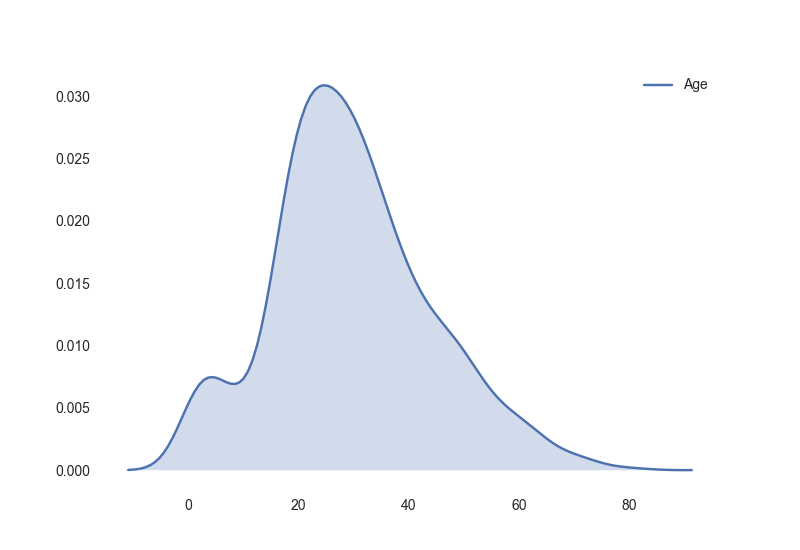#### Seaborn FacetGrid

FacetGrid is another word for subset. A powerful tool allows to visualize subsets of data according to values of another columns. See the example

``````g = sns.FacetGrid(titanic, col="Pclass", size=3);
sns.despine(left=True, bottom=True)
``````
``````<IPython.core.display.Javascript object>
``````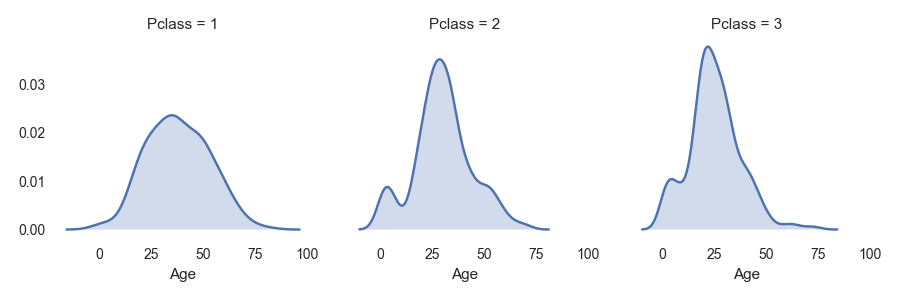Can be splitted in even more subsets (col, row and hue)

``````g = sns.FacetGrid(titanic, col="Survived", row="Pclass", hue="Sex", size=3)
sns.despine(left=True, bottom=True)
plt.show()
``````
``````<IPython.core.display.Javascript object>
``````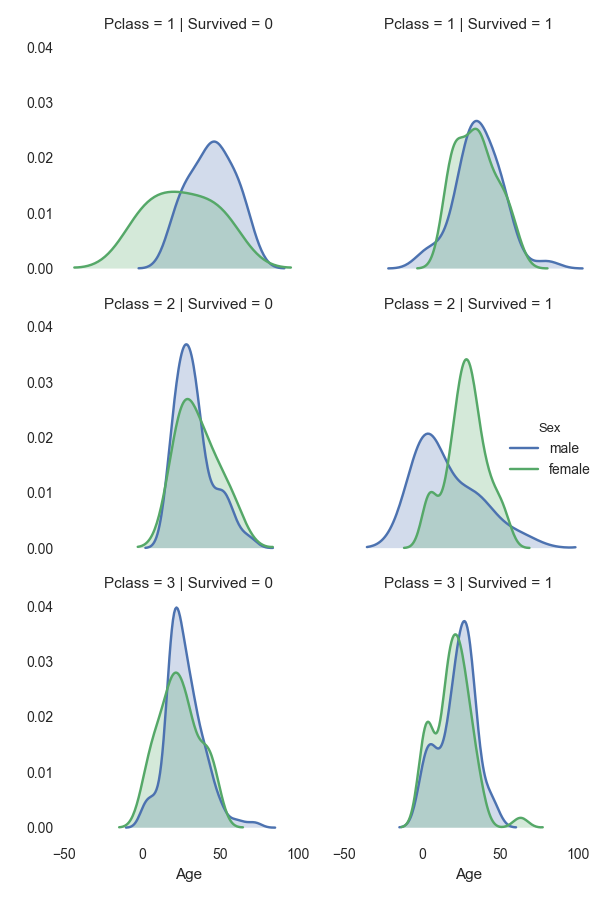Commands, snippets that might be useful for day-to-day tunes

``````# Set aspect ratio of the Artist
plt.axes().set_aspect('equal')

# Get bar rectangles
bars = [rect for rect in ax.get_children() if isinstance(rect, matplotlib.patches.Rectangle)]
``````